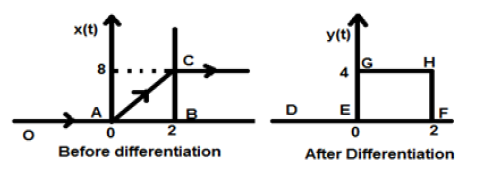# DSP - Operations on Signals Differentiation

#### Digital Marketing Online Training

23 Lectures 2 hours

#### How To Start Your Own Digital Marketing Agency

125 Lectures 9 hours

#### Digital Marketing Agency Elite Consultants Masterclass

140 Lectures 20 hours

Two very important operations performed on the signals are Differentiation and Integration.

## Differentiation

Differentiation of any signal x(t) means slope representation of that signal with respect to time. Mathematically, it is represented as;

$$x(t)\rightarrow \frac{dx(t)}{dt}$$

In the case of OPAMP differentiation, this methodology is very helpful. We can easily differentiate a signal graphically rather than using the formula. However, the condition is that the signal must be either rectangular or triangular type, which happens in most cases.

Original Signal Differentiated Signal
Ramp Step
Step Impulse
Impulse 1

The above table illustrates the condition of the signal after being differentiated. For example, a ramp signal converts into a step signal after differentiation. Similarly, a unit step signal becomes an impulse signal.

### Example

Let the signal given to us be $x(t) = 4[r(t)-r(t-2)]$. When this signal is plotted, it will look like the one on the left side of the figure given below. Now, our aim is to differentiate the given signal.

To start with, we will start differentiating the given equation. We know that the ramp signal after differentiation gives unit step signal.So our resulting signal y(t) can be written as;

$y(t) = \frac{dx(t)}{dt}$

$= \frac{d4[r(t)-r(t-2)]}{dt}$

$= 4[u(t)-u(t-2)]$

Now this signal is plotted finally, which is shown in the right hand side of the above figure.